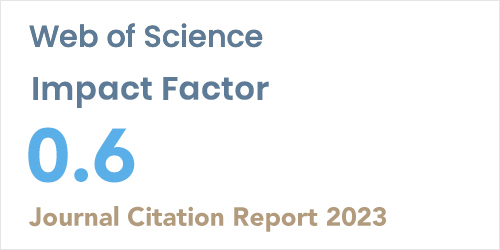ISSN: 1304-7191 | E-ISSN: 1304-7205
Volume: 41 Issue: 4 Year: 2023An introduction to zero-divisor graphs of a commutative multiplicative hyperring
1Department of Mathematics, University of Mazandaran, Babolsar-IRAN
2School of Mathematics, University of Tehran, Tehran-IRAN
Sigma J Eng Nat Sci 2018; 9(): 101-106

#### Abstract

The purpose of this paper is the study of zero-divisor graphs of a commutative multiplicative hyperrings, as a generalization of commutative rings. In this regards we consider a commutative multiplicative hyperring (𝑅,+,𝑜), where (𝑅,+) is an abelian group, (𝑅,+) is a semihypergroup and for all 𝑎,𝑏,𝑐 ∈ 𝑅, :𝑎 𝑜 (𝑏 + 𝑐) ⊆ 𝑎 𝑜 𝑏 + 𝑎 𝑜 𝑐 and (𝑎 + 𝑏) 𝑜 𝑐 ⊆ 𝑎 𝑜 𝑏 + 𝑎 𝑜 𝑐. For 𝑎 ∈ 𝑅 a nonzero element 𝑎 ∈ 𝑅 is said to be a zero-divisor of 𝑎, if 0 ∈ 𝑎 𝑜 𝑏 and the set of zero-divisors of 𝑅 is denoted by 𝑍(𝑅). We associative to 𝑅 a zero-divisor graph 𝛤(𝑅), whose vertices of 𝛤(𝑅) are the elements of 𝑍(𝑅)∗(= Z(R)\{0}) and two distinct vertices of 𝛤(𝑅) are adjacent if they were in 𝑍(𝑅). Finally, we obtain some properties of 𝛤(𝑅) and compare some of its properties to the zero-divisor graph of a classical commutative ring and show that almost all properties of zero-divisor graphs of a commutative ring can be extend to 𝛤(𝑅) while 𝑅 is a strongly distributive multiplicative hyperring.